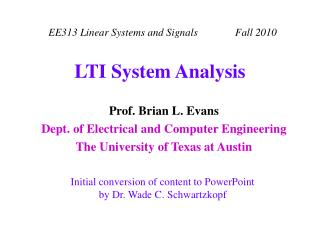Download PresentationLTI System Analysis

# LTI System Analysis - PowerPoint PPT Presentation

LTI System Analysis. Zero-State LTIC System Response. LTIC: Linear Time-Invariant Continuous-time System response = zero-input response + zero-state response Approaches to find the zero-state response Time-domain solutions to the differential equationI am the owner, or an agent authorized to act on behalf of the owner, of the copyrighted work described.
Download Presentation## LTI System Analysis

Download Policy: Content on the Website is provided to you AS IS for your information and personal use and may not be sold / licensed / shared on other websites without getting consent from its author.While downloading, if for some reason you are not able to download a presentation, the publisher may have deleted the file from their server.

- - - - - - - - - - - - - - - - - - - - - - - - - - E N D - - - - - - - - - - - - - - - - - - - - - - - - - -
Presentation Transcript
1. LTI System Analysis

2. Zero-State LTIC System Response • LTIC: Linear Time-Invariant Continuous-time • System response =zero-input response + zero-state response • Approaches to find the zero-state response Time-domain solutions to the differential equation Convolution in time domain: y(t) = f(t) * h(t) Continuous-time Fourier transform: Y(w) = F(w) H(w) Laplace transform: Y(s) = F(s) H(s)

3. Example #1 • Transfer function1/(s+2) and input e -t u(t) • Either way takes about the same amount of work

4. Example #2: Laplace is Easier • Transfer function1/(s+2) and input u(t)

5. Example #3: Laplace Cannot Work • Transfer function1/(s+2) and input et u(-t)

6. Signal Distortion • Total distortion Y(w) = F(w) H(w) H(w) is the frequency response • Magnitude distortion |Y(w)| = |F(w)| |H(w)| • Phase distortion Y(w) = (F(w) H(w)) = F(w) + H(w) • All-pass filter: magnitude response of 1 Example: Phase shifting by 90° to convert cos(2pfct) into sin(2pfct) known as a Hilbert transformer

7. Distortionless Transmission • Want distortionless transmission: input and output signals have identical shapes, differ by a multiplicative constant, and may be delayed y(t) = k f(t - t) Y(w) = k e - j w t F(w) H(w) = Y(w)/F(w) = k e - j w t Magnitude response: |H(w)| = k Phase response (linear):  H(w) = – w t Time delay is the negative of the derivative of H(w) w/r to w • Channels have distortion Receiver needs to know the channel distortion Receiver uses an equalizer.

8. Speech signals Use phase differences to locate a speaker Once locked onto a speaker, our ears are relatively insensitive to phase distortion in speech from that speaker (underlies speech compression systems, e.g. digital cell phones.) Linear phase crucial Audio Images Communication systems Linear phase response Need FIR filters Realizable IIR filters cannot achieve linear phase response Importance of Linear phase